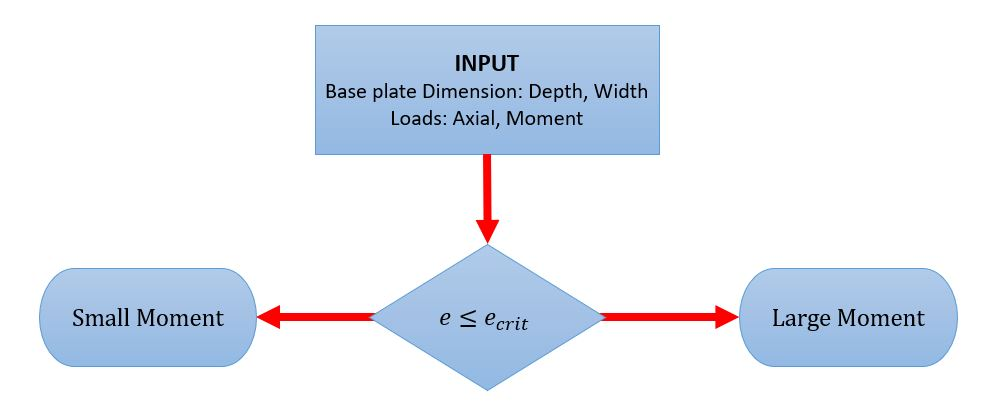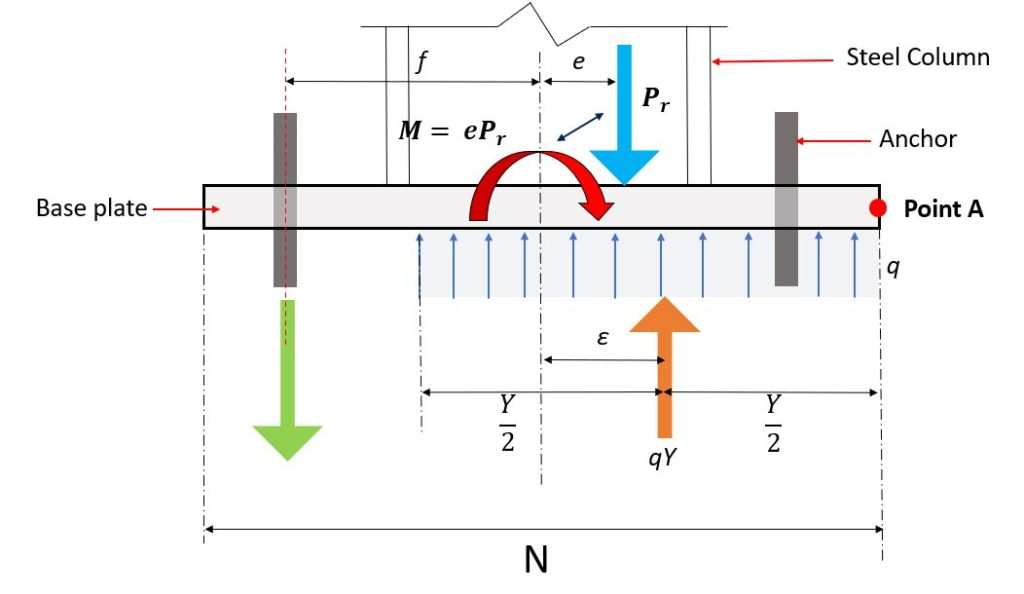Documentação SkyCiv

Seu guia para o software SkyCiv - tutoriais, guias de instruções e artigos técnicos

1. Casa
2. Projeto de conexão SkyCiv
3. Placa de base de aço (Placa de base de aço)

# Placa de base de aço (Placa de base de aço)

### Design of Steel Base Plates with Small Moment

SkyCiv Placa de base de aço adopts the design approach modified design approach method consistent with the equations of static equilibrium and the LRFD method from modified by Doyle and Fisher (2005) and considers two type of base plate with moment:

1. Small Momentthe external loads is resisted by bearing only
2. Large Momentthe external loads is resisted by both anchor roads and bearing.

### Experimente a Calculadora de Placa Base SkyCiv Gratuitamente:

General Design Procedure based AISC DG No. 1Figura 1. Steel Base Plate Design Work Flow

1. Input the Axial load and moment at SkyCiv Placa de base de aço.
2. Input the base plate size at SkyCiv Placa de base de aço

$$UMA_{1} = N \times B$$

3. The module with calculates the equivalent eccentricity,

$$e = frac{M}{N}$$

and the critical eccentricity,

$$e_{crit} = frac{N}{2} – \fratura{ a pressão exercida na parede segue uma distribuição vertical uniforme{r} }{ 2 \times q_{max} }$$

4. The module with calculates the bearing length, Y.

5. The module with calculates the required minimum base plate thickness $$tp_{req}$$.

6. The module with calculates the anchor rod size (if required).

Design Steel Base Plate with Small Moments.

O SkyCiv Placa de base de aço considers the force diagram shown in Figure 2.Figura 2. Steel Base Plate Equilibrium diagram for Small Momemts

The bearing upward force $$q$$ computes in the equation below:

$$q = f_{p} \times B$$

Onde:
$$f_{p}$$ = Bearing stress between the plate and concrete
$$B$$ = Base Plate width

The force acts at the midpoint of bearing area, ou $$\fratura{Y}{2}$$ to the left of point A. The distance of the resultant to the right of the centerline of the plate, e, é, Portanto

$$ε = \frac{N}{2} – \fratura{Y}{2}$$

It is clear that as the dimension Y decreases, ε increases. Y will reach its smallest value when q reaches its maximum:

$$Y_{min} = frac{ a pressão exercida na parede segue uma distribuição vertical uniforme{r} }{ q_{max} }$$

The expression, for the location of the resultant bearing force given in Equation 3.3.2 shows that ε reaches its maximum value when Y is minimum. Portanto

$$ε_{max} = frac{N}{2} – \fratura{ Y_{min} }{ 2 } = frac{N}{2} – \fratura{ a pressão exercida na parede segue uma distribuição vertical uniforme{você} }{ 2 \times q_{max} }$$

For moment equilibrium, the line of action of the applied load, Pu, and that of the bearing force, qY must coincide; enquanto a tensão no aço fs é menor que a tensão de escoamento f, e = ε.

$$e = frac{M}{N}$$

exceeds the maximum value that ε can attain, the applied loads cannot be resisted by bearing alone and anchor rods will be in tensionAlbert Pamonag, são influenciados principalmente pelas dimensões da sapata
Engenheiro estrutural, Desenvolvimento de Produto

## Referências

1. Steel Design Guide Base Plate and Anchor Rod Design Second Edition. Instituto Americano de Construção em Aço, 2006.
Este artigo foi útil para você?